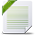# Power BI Exchange

View Only

## Forecast / Running Total#### Sam Casey Bolade11 days ago• #### 1.  Forecast / Running Total

Posted 11 days ago

Hi There,

I need a solution to this problem, please.

My dataset looks like belowSo I need to split the total budget into number of months from the start date to the end date. Which is divide (total budget, duration (months) which gives me the budget expected to spend per month.

However, I need to do a running total on this budget per month to show how much the budget should have been spent.

I used this formula below and the result isn't accumulating

Forecast Budget running total in Date =
VAR Budgetamtpermnth = DIVIDE(Sum(Sheet1[budget]),[User Duration Months])

VAR Calc=
CALCULATE(
Budgetamtpermnth ,
FILTER(
ALLSELECTED('DateTable'[Date]),
'DateTable'[Date] <= MAX('DateTable'[Date]) )
)

Return Calc------------------------------
------------------------------

Attachment(s)Forecast.pbix   87 KB 1 version

• #### 2.  RE: Forecast / Running Total

Posted 7 days ago
HI,

First, create 2 relationships between date table and sheet1and then create the following measure
``````Cumul =
VAR _CumulStart =
CALCULATE (
SUMX (
sheet1,
Sheet1[budget] / DATEDIFF ( Sheet1[Start Date], Sheet1[End Date], MONTH )
),
FILTER (
ALLSELECTED ( 'DateTable'[Date] ),
'DateTable'[Date] <= MAX ( 'DateTable'[Date] )
)
)
VAR _CumulEnd =
CALCULATE (
SUMX (
sheet1,
Sheet1[budget] / DATEDIFF ( Sheet1[Start Date], Sheet1[End Date], MONTH )
),
FILTER (
ALLSELECTED ( 'DateTable'[Date] ),
'DateTable'[Date] <= MAX ( 'DateTable'[Date] )
),
USERELATIONSHIP ( Sheet1[End Date], DateTable[Date] )
)
RETURN
_CumulStart - _CumulEnd​``````

Here is the resultI hope this is a good start for you

------------------------------
Nicolas MENDEZ
Data & BI consultant
Becom Consulting
------------------------------